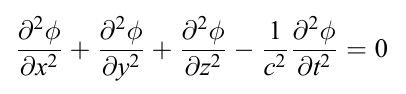### Vector Field Visualization

 the nabla symbol, ∇, denotes the three-dimensional gradient operator, del, the ∇⋅ symbol (pronounced “del dot”) denotes the divergence operator,the ∇× symbol (pronounced “del cross”) denotes the curl operator.E is the electric vector
B is the magnetic induction
ρ is the electric charge density
j is the electric current density
ε0 is the permittivity of free space
ε is the dielectric constant (or permittivity)
c is the speed of light
σ is the specific conductivity
μ is the magnetic permeability

j=σE
D=εE      D is the electric displacement
B=μH     H is the magnetic vector

### Maxwell Equations

Gauss’s law
Divergence of electric field is proportional to  the charge density inside volume

This is an abuse of notation

This has  abuse of notation

is the outward pointing unit normal at each point on every on the boundary  of surface S of Volume V

Gauss’s law for magnetism
The magnetic field B has divergence equal to zero. It is equivalent to the statement that magnetic monopoles do not exist.

This is an abuse of notation

A circulating electric field is produced by a magnetic field that changes with time.

Curl of E , the circulation of electric field , around an axis perpendicular to each plane at point (x,y,z) is equal to the rate of change in magnetic field

This is an abuse of notation

Ampere-Maxwell Law
A circulating magnetic field is produced by an electric current and by an electric field that changes with time.

This is an abuse of notation

Curl of B , the circulation of magnetic  field , around an axis perpendicular to each plane at point (x,y,z) is proportional  to the rate of change in Electric field + electrical current

if  J=0 thenhttps://web.mit.edu/sahughes/www/8.022/lec20.pdf

https://www.fiberoptics4sale.com/blogs/electromagnetic-optics/a-plain-explanation-of-maxwells-equations#:~:text=4.-,Ampere-Maxwell’s%20Law,field%20that%20changes%20with%20time.

https://en.wikipedia.org/wiki/Displacement_current

https://physics.stackexchange.com/questions/166888/the-propagation-of-electric-field

### Liénard–Wiechert potential classical electromagnetic effect of a moving electric point charge

Feynman’s derivation of Liénard-Wiechert potentials revisited

In Farsi
میدان و امواج
الکترومغناطیس ١

### Feynman

Richard P Feynman: Quantum Mechanical View of Reality – YouTube

### Explanations

This relates to electric field in that the charge moving through a circuit to light a light bulb has to be driven by some electric field, so you can reasonably ask how that field is established, and how much time it takes. Qualitatively, the necessary field is established by excess charge on the surface of the wires, with the surface charge being generally positive near the positive terminal of a battery and generally negative near the negative terminal, and dropping off smoothly from one to the other so that the electric field is more or less piecewise constant (that is, the field is the same everywhere inside a wire, and the field is the same everywhere inside a resistor, but the two field values are not the same).

When the circuit is first connected, there is a rapid redistribution of the charge on the surface of the wires which establishes the surface charge gradients that drive the steady-state current that will eventually do whatever it is you want it to do. The time required to establish the gradients and settle in to the steady-state condition is very fast, most likely on the order of nanoseconds for a normal circuit.

https://physics.stackexchange.com/questions/1747/is-there-a-travelling-speed-of-for-electric-field-if-yes-what-is-it

Since 11 April 2023: 207 total views,  1 views today